# The Universe of Discourse

Mon, 17 Aug 2015

This is page 4 of the Cosmic Call message. An explanation follows.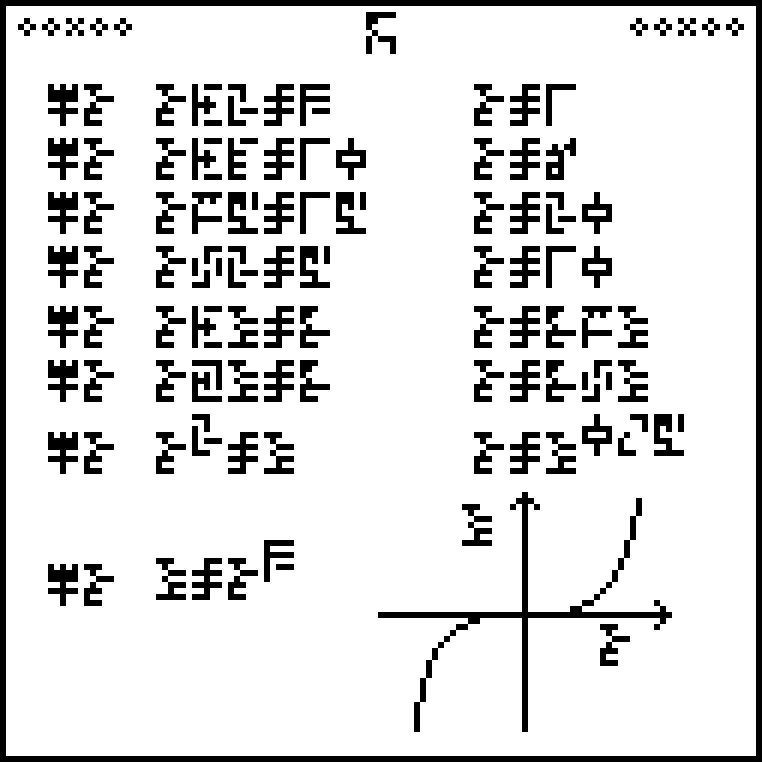Reminder: page 1 explained the ten digits: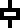0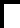1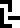2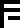3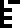4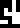5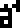6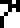7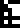8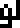9

And the equal sign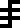. Page 2 explained the four basic arithmetic operations and some associated notions: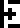addition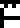subtraction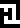multiplication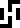division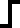negation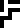ellipsis (…)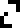decimalpoint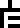indeterminate

This page, headed with the glyph for “mathematics”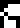, describes the solution of simple algebraic equations and defines glyphs for three variables, which we may as well call !!x,y,!! and !!z!!: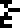x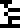y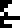z

Each equation is introduced by the locution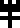which means “solve for !!x!!”. This somewhat peculiar “solve” glyph will not appear again until page 23.

For example the second equation is !!x+4=10!!: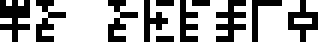Solve for !!x!!: !!x+4=10!!

The solution, 6, is given over on the right: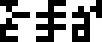!!x=6!!

After the fourth line, the equations to be solved change from simple numerical equations in one variable to more abstract algebraic relations between three variables. For example, if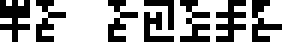Solve for !!x!!: !!x\cdot y=z!!

then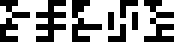!!x=z\div y!!.

The next-to-last line uses a decimal fraction in the exponent, !!0.5!!: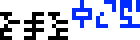. On the previous page, the rational fraction !!1\div 2!! was used. Had the same style been followed, it would have looked like this: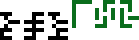.

Finally, the last line defines !!x=y^3!! and then, instead of an algebraic solution, gives a graph of the resulting relation, with axes labeled. The scale on the axes is not the same; the !!x!!-coordinate increases from 0 to 20 pixels, but the !!y!!-coordinate increases from 0 to 8000 pixels because !!20^3 = 8000!!. If axes were to the same scale, the curve would go up by 8,000 pixels. Notice that the curve does not peek above the !!x!!-axis until around !!x=8, y=512!! or so. The authors could have stated that this was the graph of !!y=x^3\div 400!!, but chose not to.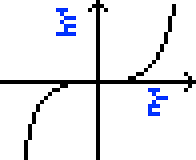I also wonder what the aliens will make of the arrows on the axes. I think the authors want to show that our coordinates increase going up and to the left, but this seems like a strange and opaque way to do that. A better choice would have been to use a function with an asymmetric graph, such as !!y=2^x!!.

(After I wrote that I learned that similar concerns were voiced about the use of a directional arrow in the Pioneer plaque.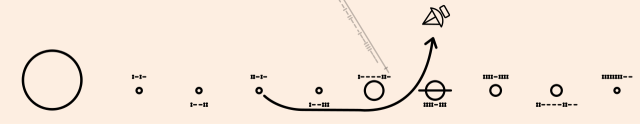(Wikipedia says: “An article in Scientific American criticized the use of an arrow because arrows are an artifact of hunter-gatherer societies like those on Earth; finders with a different cultural heritage may find the arrow symbol meaningless.”)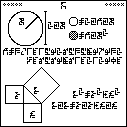The next article will discuss page 5, shown at right. (Click to enlarge.) Try to figure it out before then.